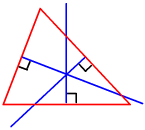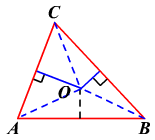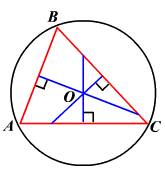# Circumcenter Theorem

### Circumcenter

The three perpendicular bisectors of a triangle meet in a single point, called the circumcenter .### Circumcenter Theorem

The vertices of a triangle are equidistant from the circumcenter.

Given:

$\Delta ABC$ , the perpendicular bisectors of $\stackrel{¯}{AB},\stackrel{¯}{BC}$ and $\stackrel{¯}{AC}$ .

To prove:

The perpendicular bisectors intersect in a point and that point is equidistant from the vertices.The perpendicular bisectors of $\stackrel{¯}{AC}$ and $\stackrel{¯}{BC}$ intersect at point $O$ .

Let us prove that point $O$ lies on the perpendicular bisector of $\stackrel{¯}{AB}$ and it is equidistant from $A$ , $B$ and $C$ .

Draw $\stackrel{¯}{OA},\stackrel{¯}{OB}$ and $\stackrel{¯}{OC}$ .

Any point on the perpendicular bisector of a segment is equidistant from the endpoints of the segment.

So, $OA=OC$ and $OC=OB$ .

By the transitive property,

$OA=OB$ .

Any point equidistant from the end points of a segment lies on its perpendicular bisector.

So, $O$ is on the perpendicular bisector of $\stackrel{¯}{AB}$ .

Since $OA=OB=OC$ , point $O$ is equidistant from $A$ , $B$ and $C$ .This means that there is a circle having its center at the circumcenter and passing through all three vertices of the triangle.  This circle is called the circumcircle .